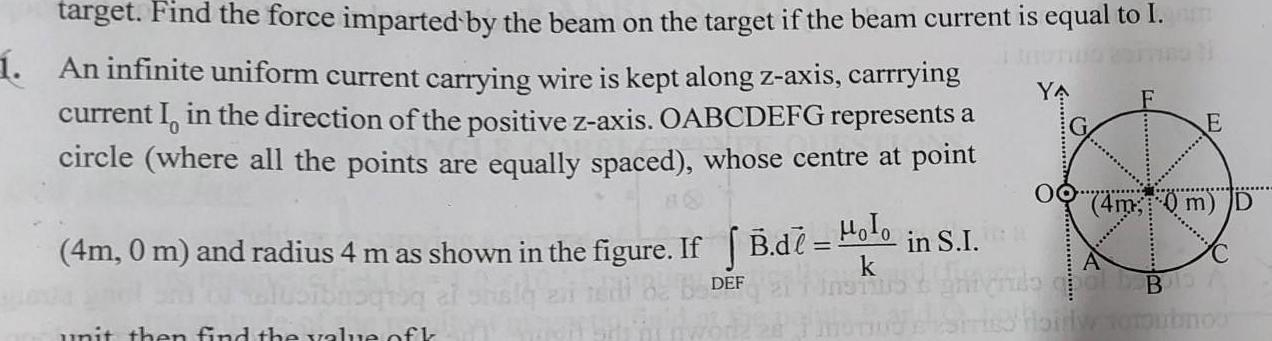Question:

# target Find the force imparted by the beam on the target if

Last updated: 6/2/2023target Find the force imparted by the beam on the target if the beam current is equal to I 1 An infinite uniform current carrying wire is kept along z axis carrrying current I in the direction of the positive z axis OABCDEFG represents a circle where all the points are equally spaced whose centre at point 4m 0 m and radius 4 m as shown in the figure If B d DEF unit then find the value of k YA E 00 4m 0 m D A Holo in S I A shus grinde OLD BIS A Stries Horlw tomoubnoo